# Constructions Class 9

In Constructions class 9 chapter 11, explains how different shapes are drawn using the compass and ruler. In this chapter, you can learn how to construct the bisector of the given angle, construction of a perpendicular bisector of the given line segment are explained with construction steps and clear diagram. Also, solve all the important questions for class 9 Maths Chapter 11 construction problems given in the textbook to score good marks.

## Constructions Class 9 Concepts

The important topics and subtopics covered under constructions class 9 are:

• Introduction
• Basic constructions
• Some constructions of triangles
• Summary

## Construction Class 9 Notes

For constructing the angle bisector of angle ABC, take B as a centre construct an arc (any radius) intersecting the rays BA and BC at D and E. Now take E and D as centres and radius more than ½ DE, construct arcs such that they intersect with each other at F.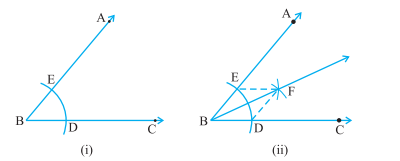Join BF. This line is the required angle bisector of given angle ABC.

Proof:

Join EF and DF. Now, from triangle BEF and triangle BDF, BD = BE (Radius of the same arc), DF = EF (Arcs of equal radii) and BF is Common. Therefore, triangle BEF is congruent to triangle BDF by SSS congruence rule. Hence by CPCT, angle EBF is equal to angle DBF.

For constructing the perpendicular bisector of a line segment AB. Take A and B as centres and radius greater than ½ AB. Draw arcs on both sides of AB making them intersect at Q and P. Join QP. Let QP intersect BA at M. Then, QMP is the required perpendicular bisector of BA.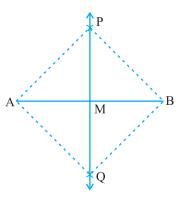Proof: Consider triangles QAP and QBP,

PA = PB, QA = QB (Arcs of equal radii) and PQ is Common. Therefore, triangle PAQ is congruent to triangle PBQ by SSS congruence rule. Therefore, by CBCT, angle APM is equal to angle BPM Now, consider triangles AMP and BMP, PA = PB, PM is Common, and angle MPA = angle MPB (Proved). Therefore, triangle PMA is congruent to triangle PMB by SAS congruence rule. Therefore, by CPCT MA = MB and angle AMP = angle BMP As angle AMP + angle BMP = 180 degree therefore, angle PMA = angle PMB = 90°.

### Constructions Class 9 Examples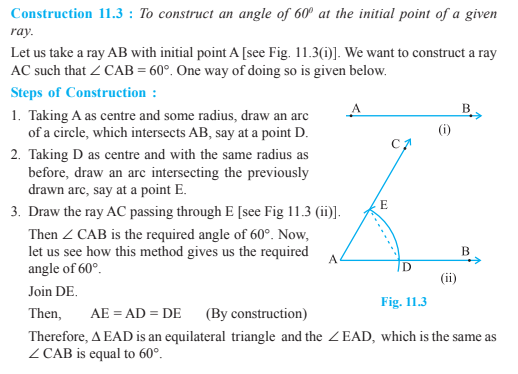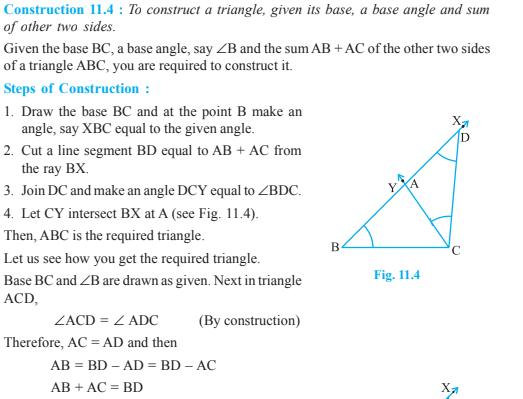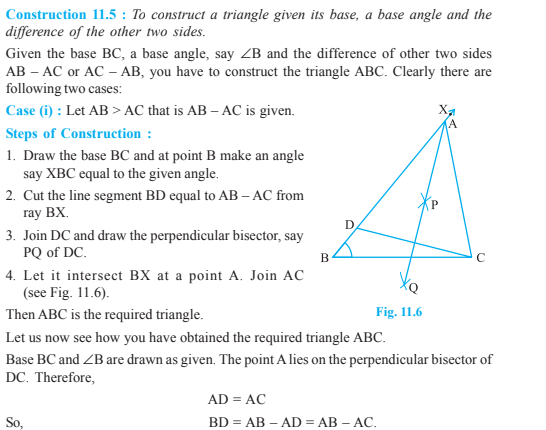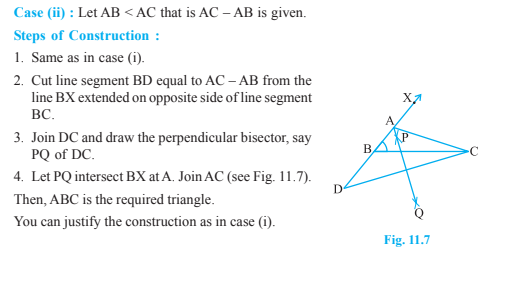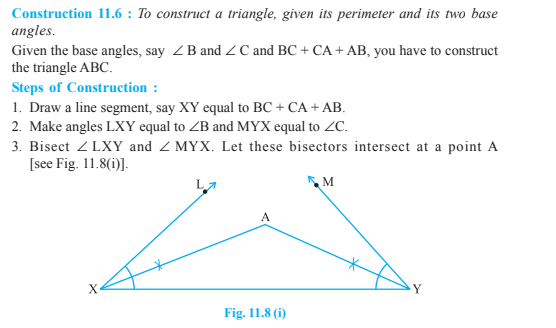For more information on class 9 concepts and notes, register with BYJU’S – The Learning App and also refer NCERT, RD Sharma, RS Agarwal solutions to solve important questions.